Scroll

# Programming Col Sig (proportions): Part 4 - (Student Test using estimator & efficiency coefficient)

Follow
 Summary This article demonstrates how to script the formula for Student Test using efficiency coefficient on closed questions. Formula and syntax are shown. There is also a demonstration of calculating Effective base manually. Applies to Askia Analyse. Written for Researchers, Developers, Data Processors. Keywords Table arithmetic, Forloops, Coordinates, data, Calculation arithmetic, Bespoke calculation, Filtered, Multiplication table, Classical Student Test, Sigma2, Sigma3, Z-test, Unpooled variance, Confidence interval, 95%, FilterBy, GetColSigLetter, Effective Base, Weighting, Generate intermediary results, DebugTrace, DebugColSig.

The .qes file and portfolio containing the examples discussed is attached: Table Arithmetic Examples 19.rar.

Student Test using estimator & efficiency coefficient (Sigma4)

In this demonstration I will create a table which shows the pre-set colsig calculation side by side with the programmed calculation for the same test. First, let's review the formula: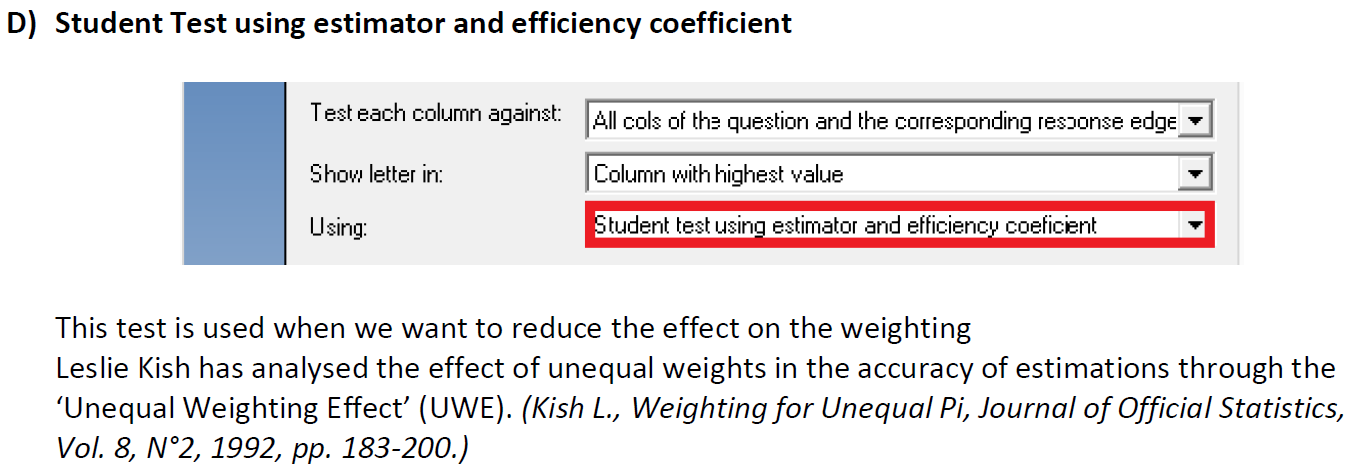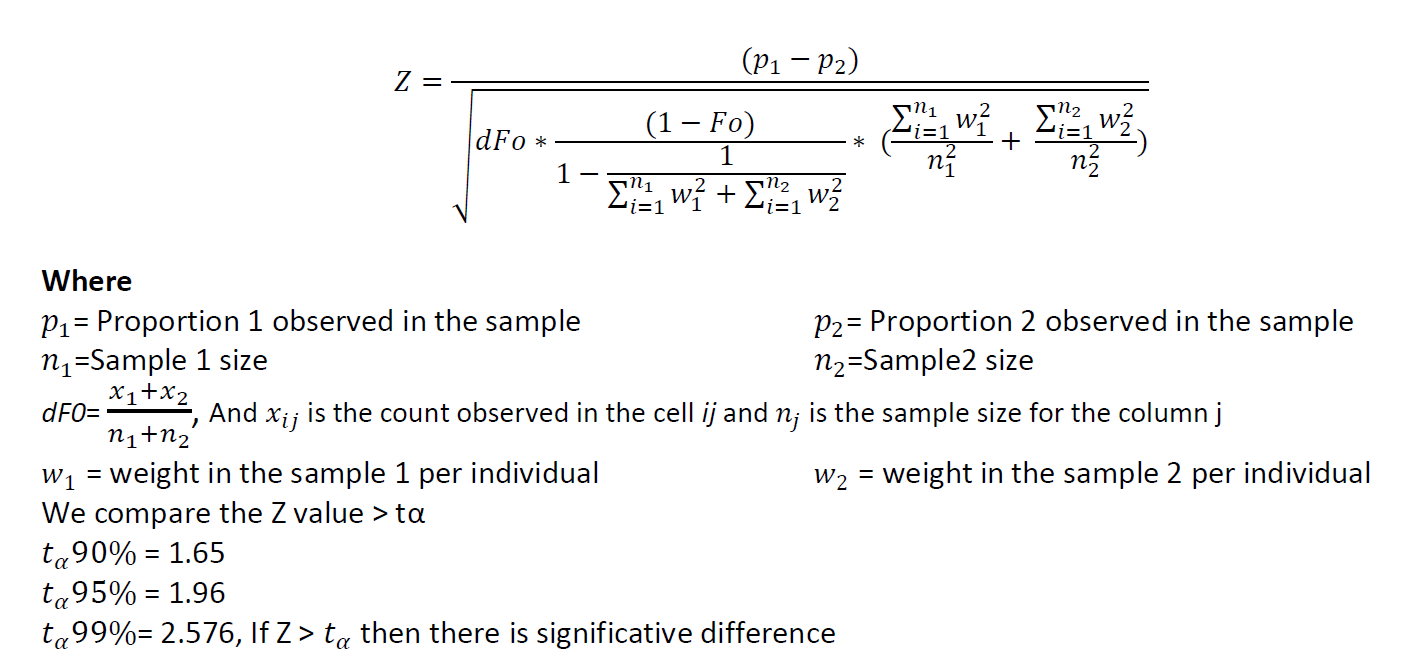You need a similar initial set-up to programming Sigma3. It's worth reading that article if you haven't already as it has a lot of useful additional detail.

Create a variable based on your weighting: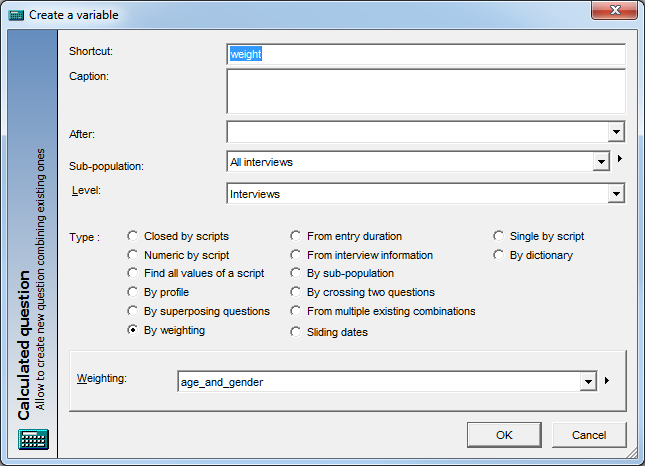Create a second variable ‘weight sq’ which is the square of weighting factors stored in this variable, ‘weight’.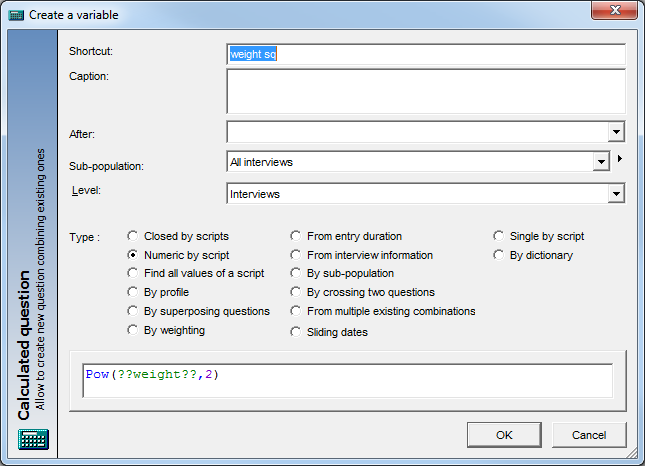Note the calculations applied: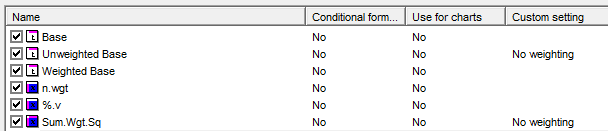Sum.Wgt.Sq is a Script (sum) calculation based on our squared weighting factors: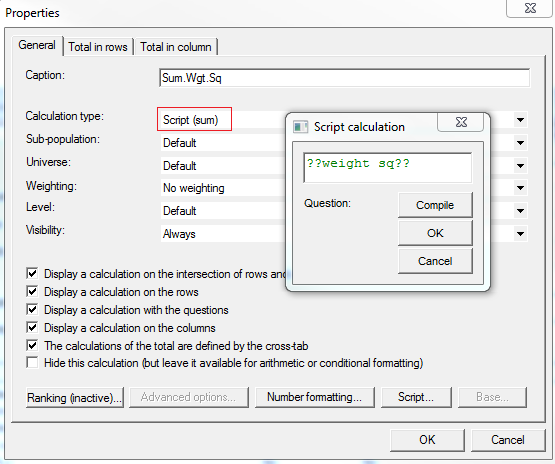There are 3 ColSig calculations.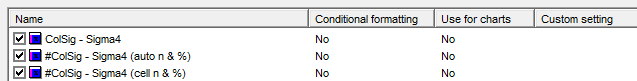ColSig - Sigma4: This is the inbuilt Col Sig calculation for student test with estimator & efficiency coefficient.

#ColSig - Sigma4 (auto n & %): This is the syntax to program the col sig using automatic count and % definitions i.e. FilterbyX,Y,XY keywords.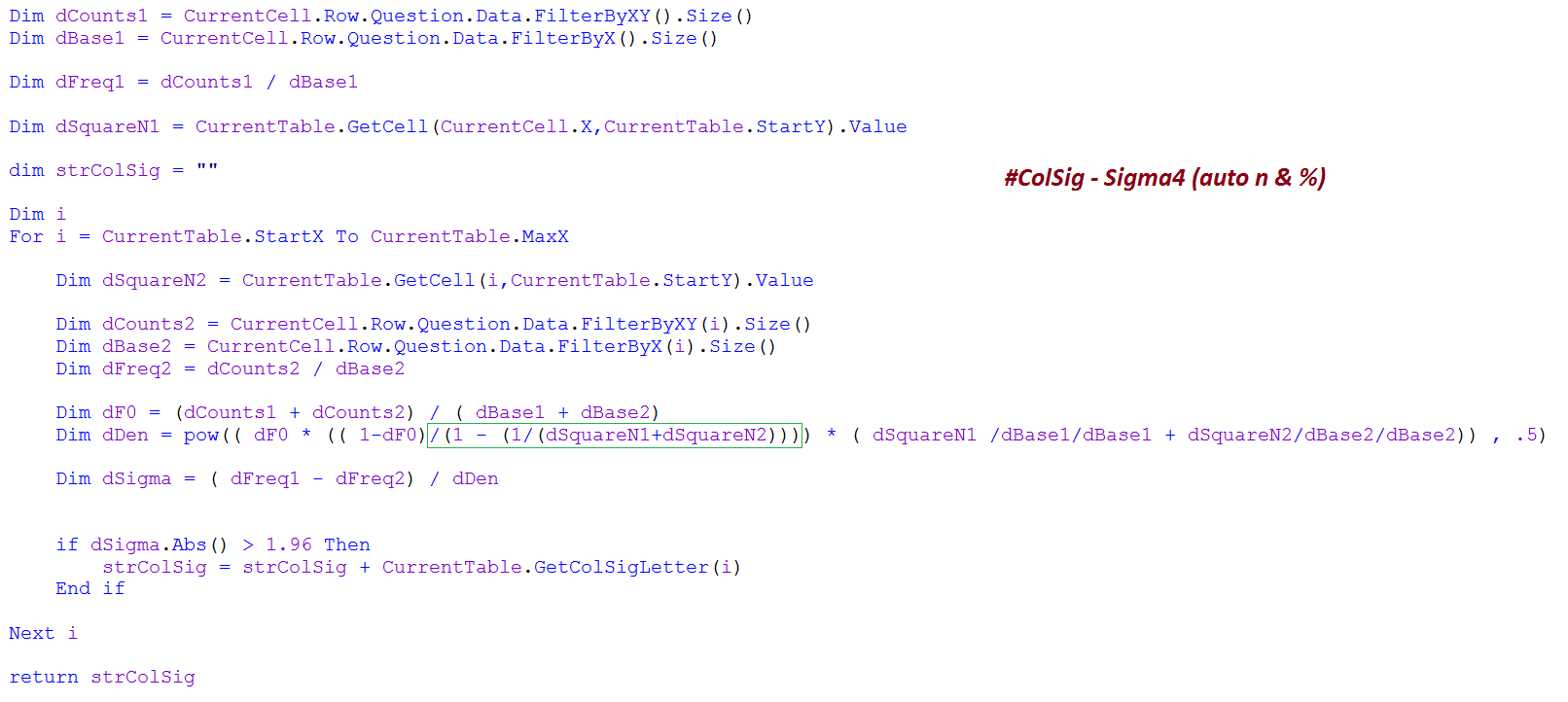#ColSig - Sigma4 (cell n & %): This is the syntax to program the col sig using count and % values shown in the current table i.e. refer to cell positions of these in relation to the current cell's y-coordinate or data start positions's y-coordinate. (See highlighted yellow sections below).

This approach will be useful if... your current table is a result of using some arithmetic or script already e.g. if my current table has figures for some brands multiplied / corrected by a factor of 0.5, and for others brands, 0.75 then the colsig using FilterbyX,Y,XY will not work as these keywords return the natural counts and bases from the variables in the cross tab, not taking notice of any corrections or manipulation applied. It's less dynamic but the benefit is that you can access the 'surface' or 'post manipulation'  values of your table. The introduction to programming Sigma1 also explains this.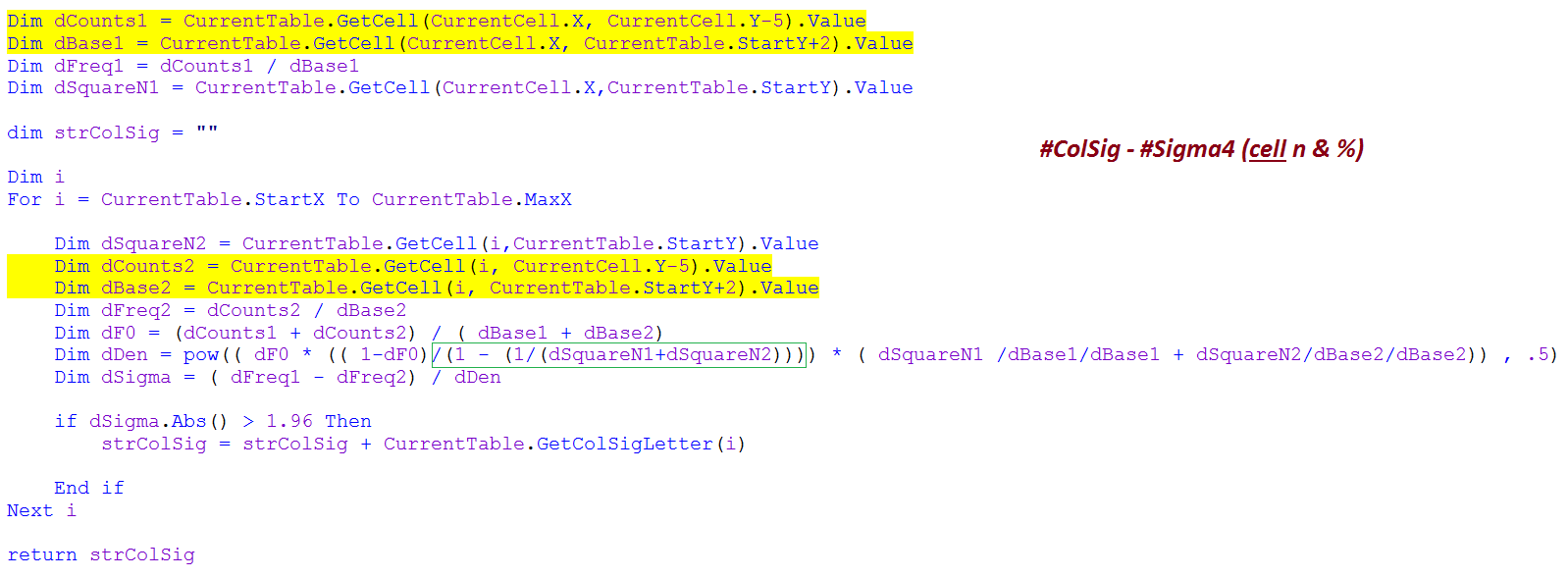The green boxed section in the syntax is the only difference required between programming Sigma4 and Sigma3.

A reminder of what the terms Sigma1 to Sigma 4 refer to: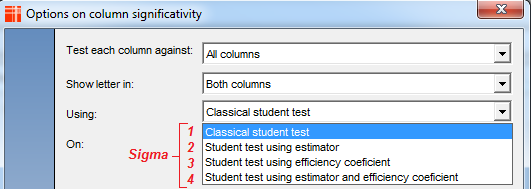It's recommended that you read the articles for the other Sigma calculations as they contain useful background (especially Sigma1 & Sigma3).